# NCERT Solutions for Class 12 Physics Chapter 3 Current Electricity in Hindi

## NCERT Solutions for Class 12 Physics Chapter 3 Current Electricity In Hindi Medium

Download the Class 12 Physics NCERT Solutions in Hindi medium and English medium as well offered by the leading e-learning platform Vedantu. If you are a student of Class 12, you have reached the right platform. The NCERT Solutions for Class 12 Physics in Hindi provided by us are designed in a simple, straightforward language, which are easy to memorise. You will also be able to download the PDF file for NCERT Solutions for Class 12 Physics  in Hindi from our website at absolutely free of cost.

NCERT, which stands for The National Council of Educational Research and Training, is responsible for designing and publishing textbooks for all the classes and subjects. NCERT textbooks covered all the topics and are applicable to the Central Board of Secondary Education (CBSE) and various state boards.

We, at Vedantu, offer free NCERT Solutions in English medium and Hindi medium for all the classes as well. Created by subject matter experts, these NCERT Solutions in Hindi are very helpful to the students of all classes.

Do you need help with your Homework? Are you preparing for Exams?
Study without Internet (Offline)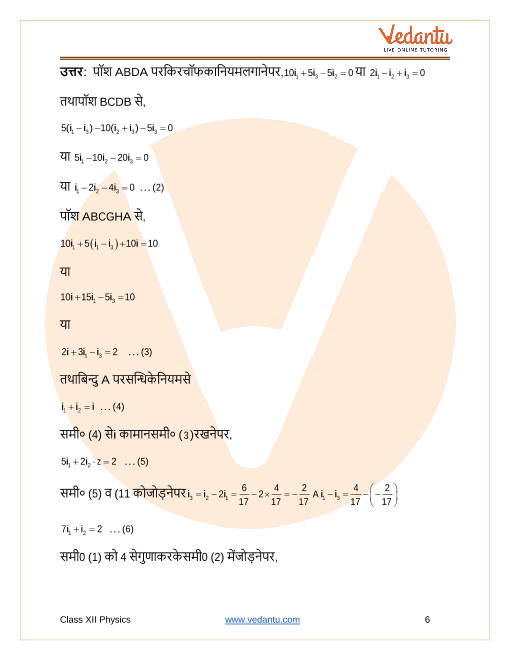## Access NCERT Solutions for class 12 Physics Chapter 3 – Current Electricity

### अभ्यास के अन्तर्गत दिए गए प्रश्नोत्तर

1.किसी कार की संचायक बैटरी का विद्युत वाहक बल ${\mathbf{12}}{\text{ }}{\mathbf{V}}$है। यदि बैटरी को आन्तरिक प्रतिरोध ${\mathbf{0}}.{\mathbf{4}}{\text{ }}\Omega$हो तो बैटरी से ली जाने वाली अधिकतम धारा का मान ज्ञात कीजिए?

हल-$E$वैद्युत वाहक बल वाली बैटरी से ली जाने वाली धारा होगी ,

$I = \frac{E}{{R + r}}$

जिसमें $R$बाह्य प्रतिरोध तथा $r$आन्तरिक प्रतिरोध है।

अधिकतम धारा के लिए बाह्य प्रतिरोध होगा , $R{\text{ }} = {\text{ }}0$

धारा,$I = \frac{E}{r} = \frac{{12}}{{0.4}} = 30A$

2.${\mathbf{10}}{\text{ }}{\mathbf{V}}$विद्युत वाहक बल वाली बैटरी जिसका आन्तरिक प्रतिरोध ${\mathbf{3}}{\text{ }}\Omega$है, किसी प्रतिरोधक से संयोजित है। यदि परिपथ में धारा का मान ${\mathbf{0}}.{\mathbf{5}}{\text{ }}{\mathbf{A}}$हो तो प्रतिरोधक का प्रतिरोध क्या होगा है?जब परिपथ बन्द है ,तो सेल की टर्मिनल वोल्टता क्या होगी?

हल-दिया है, बैटरी का वैद्युत वाहक बल $E{\text{ }} = {\text{ }}10$$V$

बैटरी का आन्तरिक प्रतिरोध $= {\text{ }}3$ $\Omega$

| परिपथ में धारा $I{\text{ }} = {\text{ }}0.5$$A$

प्रतिरोधक का प्रतिरोध $R{\text{ }} = {\text{ }}?$

बन्द परिपथ में बैटरी की टर्मिनल वोल्टता $V = {\text{ }}?$

सूत्र $I = \frac{E}{{R + r}}$ से

$R + r\;\; = \frac{E}{I}$

बैटरी की टर्मिनल वोल्टता $V = E - Ir$

$= 10 - 0.5 \times 3\; = 10 - 1.5 = 8.5\;\;V$

3.

1. ${\mathbf{1}}{\text{ }}\Omega ,{\text{ }}{\mathbf{2}}{\text{ }}\Omega$और ${\mathbf{3}}{\text{ }}\Omega$के तीन प्रतिरोधक श्रेणी में संयोजित हैं। प्रतिरोधकों के संयोजन का कुल प्रतिरोध क्या है?

2. (b) यदि प्रतिरोधकों का संयोजन किसी ${\mathbf{12}}{\text{ }}{\mathbf{V}}$की बैटरी जिसका आन्तरिक प्रतिरोध नगण्य है ,से सम्बद्ध है ,तो प्रत्येक प्रतिरोधक के सिरों पर वोल्टता पात ज्ञात कीजिए।

हल-दिया है, ${R_1} = {\text{ }}1{\text{ }}\Omega ;{\text{ }}{R_2} = {\text{ }}2{\text{ }}\Omega ;{\text{ }}{R_3} = {\text{ }}3{\text{ }}\Omega$

1. यदि श्रेणी संयोजन में तुल्य प्रतिरोध $R$हो, तो

$R{\text{ }} = {\text{ }}{R_1} + {\text{ }}{R_2} + {\text{ }}{R_3} = {\text{ }}1{\text{ }} + {\text{ }}2{\text{ }} + {\text{ }}3{\text{ }} = {\text{ }}6$$\Omega$

1. दिया है, बैटरी का वै० वा० बल $E{\text{ }} = {\text{ }}12$$V$

बैटरी का आन्तरिक प्रतिरोध $r{\text{ }} = {\text{ }}0$

तथा बाह्य प्रतिरोध $R{\text{ }} = {\text{ }}6$$\Omega$

यदि संयोजन द्वारा परिपथ में प्रवाहित धारा i हो, तो

$i\;\; = \frac{E}{{R + r}}\;\;\; = \frac{{12}}{{6 + 0}} = 2\;\;\;A$

अत: विभव पतन

${R_1}$ पर$\;{V_1}\;\; = i{R_1}\;\;\;\; = 2 \times 1 = 2\;V$

${R_2}$पर $\;{V_2}\;\; = i{R_2}\;\;\;\; = 2 \times 2 = 4\;V$

${R_3}$पर $\;{V_3}\;\; = i{R_3}\;\;\;\; = 2 \times 3 = 6\;\;V$

4.

1. ${\mathbf{2}}{\text{ }}\Omega ,{\text{ }}{\mathbf{4}}{\text{ }}\Omega$ और ${\mathbf{5}}{\text{ }}\Omega$ के तीन प्रतिरोधक पार्श्व में संयोजित हैं। संयोजन का कुल प्रतिरोध ज्ञात कीजिए?

2. यदि संयोजन को ${\mathbf{20}}{\text{ }}{\mathbf{V}}$के विद्युत वाहक बल की बैटरी जिसका आन्तरिक प्रतिरोध नगण्य है, से सम्बद्ध किया जाता है, तो प्रत्येक प्रतिरोधक से प्रवाहित होने वाली धारा तथा बैटरी से ली गई कुल धारा का मान ज्ञात कीजिए।
हल-

(a) समान्तरक्रम में तुल्य प्रतिरोध ${R_p}$के लिए

(b) समान्तरक्रम में प्रत्येक प्रतिरोध के सिरों के बीच ज्यात समान रहता है।

$\therefore \;$ प्रतिरोध ${R_1}$ में धारा, ${I_1} = \frac{V}{{{\eta _i}}} = \frac{n}{L}$ ।e $A$

प्रतिरोध ${R_2}$ में धारा, $/{z^2} = \frac{r}{{{R_2}}} = \frac{{20}}{4} = 5A$

प्रतिरोध ${R_3}$ में धारा, ${I_3} = \frac{V}{{{R_3}}} = \frac{{20}}{5} = 4\;A$

बैटरी से ली गई कुल धारा,

$I = {I_1} + {I_2} + {I_3} = 10 + 5 + 4 = 19\;A$

5.कमरे के ताप (${\mathbf{27}}.{\mathbf{0}}^\circ {\mathbf{C}}$) पर किसी तापन-अवयव का प्रतिरोध ${\mathbf{100}}{\text{ }}\Omega$है। यदि तापन-अवयव का प्रतिरोध ${\mathbf{117}}{\text{ }}\Omega$हो तो अवयव का ताप क्या होगा? प्रतिरोधक के पदार्थ का ताप-गुणांक ${\mathbf{1}}.{\mathbf{70}}{\text{ }}{\mathbf{x}}{\text{ }}{\mathbf{1}}{{\mathbf{0}}^{ - {\mathbf{4}}}}^\circ {{\mathbf{C}}^{ - {\mathbf{1}}}}\;$है।

हल- प्रश्नानुसार,

${R_{27}} = 100\Omega ,{R_T} = 117\Omega ,T = ?;\alpha = 1.70 \times {10^{ - 4}}{\left( {{\;^ \circ }C} \right)^{ - 1}}$

ताप गुणांक, $\alpha = \frac{{{R_T} - {R_{27}}}}{{{R_{27}}\left( {T - 27} \right)}}$, ताप्र ज्ञज्ञात है।

$\Rightarrow \;\;T - 27\; = \frac{{{R_T} - {R_I}}}{\lambda } \div \cdot \frac{{117 - 100}}{{100 \times 1.70 \times {{10}^{ - 4}}}} = 1000\;\;\therefore \;T\;\; - 1000 + 27 = {1027^ \circ }C\;$

6.${\mathbf{15}}$मीटर लम्बे एवं ${\mathbf{6}}.{\mathbf{0}}{\text{ }}{\mathbf{x}}{\text{ }}{\mathbf{1}}{{\mathbf{0}}^{ - {\mathbf{7}}}}\;{{\mathbf{m}}^{\mathbf{2}}}$अनुप्रस्थ काट वाले तार से उपेक्षणीय धारा प्रवाहित की गई है, और इसका प्रतिरोध ${\mathbf{5}}.{\mathbf{0}}{\text{ }}\Omega$मापा गया है। प्रायोगिक ताप पर तार के पदार्थ की प्रतिरोधकता ज्ञात कीजिए?

हल

-दिया गया है,कि  तार की लम्बाई $l{\text{ }} = {\text{ }}15\;m$

तार की अनुप्रस्थ काट का क्षेत्रफल $A{\text{ }} = {\text{ }}6.0{\text{ }} \times {10^{ - 7}}\;m$

तथा तार का प्रतिरोध $R{\text{ }} = {\text{ }}5.0\;\Omega$

तार के पदार्थ की प्रतिरोधकता ρ = ?

सूत्र, $R = \rho \frac{l}{A}$

$\begin{array}{*{20}{c}}{\rho = \frac{{RA}}{l}} \\ { = \frac{{5.0 \times 6.0\times {{10}^{ - 7}}}}{{15}}} \end{array}$

$= 2 \times {10^{ - 7}}\;\Omega m$

7. सिल्वर के किसी तार का 27.5°C प प्रतिरोध 2.1 Ω और 100°C पर प्रतिरोध 2.7 Ω है। सिल्वर का प्रतिरोधकता ताप-गुणांक ज्ञात कीजिए।

हल: प्रश्नानुसार,

$\begin{array}{*{20}{r}}{{R_1} = 2.1{{\Omega }},{t_1} = {{27.5}^ \circ }{\text{C}}} \\ {\quad {R_2} = 2.7{t{\Omega }},{t_2} = {{100}^ \circ }{\text{C}},\alpha = ?} \end{array}$

सिल्वर की प्रतिरोधकता का ताप गुणांक,

$\begin{array}{*{20}{c}}{\alpha = \frac{{{R_2} - {R_1}}}{{{R_1}\left( {{t_2} - {t_1}} \right)}} = \frac{{27 - 2.1}}{{21(100 - 27.5)}} = \frac{{0.6}}{{2.1 \times 72.5}}} \\ { = 0.0039{{\left( {^ \circ {\text{C}}} \right)}^{ - 1}}} \end{array}$

8. नाइक्रोम का एक तापन-अवयव ${\mathbf{230}}{\text{ }}{\mathbf{V}}$की सप्लाई से संयोजित है ,और ${\mathbf{3}}.{\mathbf{2}}{\text{ }}{\mathbf{A}}$की प्रारम्भिक धारा लेता है ,जो कुछ सेकेण्ड में ${\mathbf{2}}.{\mathbf{8}}{\text{ }}{\mathbf{A}}$पर स्थायी हो जाती है। यदि कमरे का ताप ${\mathbf{27}}.{\mathbf{0}}^\circ {\mathbf{C}}$है तो तापन-अवयव का स्थायी ताप क्या होगा? यदि दिए गए ताप-परिसर में नाइक्रोम का औसत प्रतिरोध का ताप-गुणांक $1.70{\text{ }} \times {\text{ }}{10^{ - 4}}^\circ {C^{ - 1}}\;$है।
हल:

-कमरे के ताप ${t_1} = {27^ \circ }C$ पर तापन-अवयव का प्रतिरोध

${R_1} =$सप्लाई वोल्टता /प्रारम्भिक धारा $= \frac{{230{\text{ V }}}}{{3.2{\text{ A }}}}$$= 71.875\; तापन-अवयव के स्थायी ताप t_2^ \circ C = ? पर तापन-अवयव का प्रतिरोध {R_2} = सप्लाई वोल्टता/ स्थायी धारा = \frac{{230}}{{2.8}} 9. चित्र ${\mathbf{3}}.{\mathbf{2}}$में दर्शाए नेटवर्क की प्रत्येक शाखा में प्रवाहित धारा का माप ज्ञात कीजिए।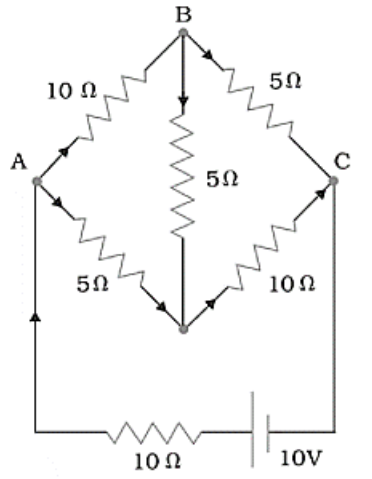हल-पॉश ABDA परकिरचॉफकानियमलगानेपर, $10{i_1} + 5{i_3} - 5{i_2} = 0$ या $2{i_1} - {i_2} + {i_3} = 0$ तथापॉश BCDB से, 5({i_1} - {i_3}) - 10({i_2} + {i_3}) - 5{i_3} = 0 या $5{i_1} - 10{i_2} - 20{i_3} = 0$ या ${i_1} - 2{i_2} - 4{i_3} = 0$ …(2) पॉश ABCGHA से, $10{i_1} + 5\left( {{i_1} - {i_3}} \right) + 10i = 10$ या $10i + 15{i_1} - 5{i_3} = 10$ या $2i + 3{i_1} - {i_3} = 2$ …(3) तथाबिन्दु A परसन्धिकेनियमसे ${i_1} + {i_2} = i$ …(4) समी० (4) सेi कामानसमी० (3)रखनेपर, $5{i_1} + 2{i_2} \cdot z = 2$ …(5) समी० (5) व (11 कोजोड़नेपर{i_3} = {i_2} - 2{i_1} = \frac{6}{{17}} - 2 \times \frac{4}{{17}} = - \frac{2}{{17}}\;A\;{i_1} - {i_3} = \frac{4}{{17}} - \left( { - \frac{2}{{17}}} \right) $7{i_1} + {i_2} = 2$ …(6) समी0 (1) को 4 सेगुणाकरकेसमी0 (2) मेंजोड़नेपर, $9{i_1} - 6{i_2} = 0\quad \Rightarrow \quad {i_2} = \frac{3}{2}{i_1}$ …(7) समी० (6) मेंमानरखनेपर, $7{i_1} + \frac{3}{2}{i_1} = 2 \Rightarrow {i_1} = \frac{{2 \times 2}}{{17}} = \frac{4}{{17}}{\text{A}}$ समी० (7) से,${i_2} = \frac{3}{2} \times \frac{4}{{17}} = \frac{6}{{17}}{\text{A}}$ = \frac{6}{{17}}\;A\;{i_2} + {i_3} = \frac{6}{{17}} - \frac{2}{{17}} = \frac{4}{{17}}\;A\;i = {i_1} + {i_2} = \frac{4}{{17}} + \frac{6}{{17}} = \frac{{10}}{{17}}\;AशाखाABमेंधारा $= 417A$ शाखा $CD\;$ मेंधा = - \frac{4}{{17}}AशाखाBCमेंधारा = \frac{6}{{17}}\;Aशाखा\;BDमेंधारा = - \frac{2}{{17}}A\; 10. 1. किसी मीटर-सेतु में जब प्रतिरोधक ${\mathbf{S}}{\text{ }} = {\text{ }}{\mathbf{12}}.{\mathbf{5}}{\text{ }}\Omega$हो तो सन्तुलन बिन्दु, सिरे ${\mathbf{A}}$से ${\mathbf{39}}.{\mathbf{5}}{\text{ }}{\mathbf{cm}}$की लम्बाई पर प्राप्त होता है। ${\mathbf{R}}$का प्रतिरोध ज्ञात कीजिए। व्हीटस्टोन सेतु या मीटर सेतु में प्रतिरोधकों के संयोजन के लिए मोटी कॉपर की पत्तियाँ क्यों प्रयोग में लाते हैं ? 2. ${\mathbf{R}}$तथा ${\mathbf{S}}$को अन्तर्बदल करने पर उपर्युक्त सेतु का सन्तुलन बिन्दु ज्ञात कीजिए। 3. यदि सेतु के सन्तुलन की अवस्था में गैल्वेनोमीटर और सेल का अन्तर्बदल कर दिया जाए तब क्या गैल्वेनोमीटर कोई धारा दर्शाएगा?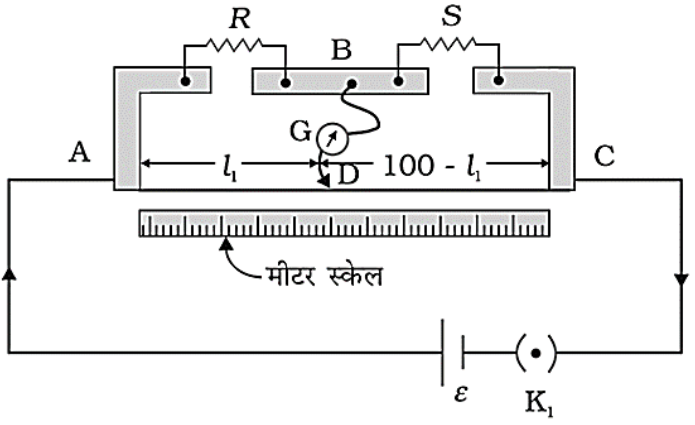हल: दिया है, 1. {l_1} = 39.5 सेमी, \;R = ?,S = 12.5\;\Omega सूत्र \frac{P}{Q} = \frac{R}{S} से, \frac{{{l_1}}}{{100 - {l_1}}} = \frac{R}{S} अथवा \begin{gathered}R = \left( {\frac{{{l_1}}}{{100 - {l_1}}}} \right) \hfill \\S = \frac{{39.5 \times 12.5}}{{\left( {100 - 39.5} \right)}} = \frac{{39.5 \times 12.5}}{{60.5}}\; \hfill \\R = 8.16\;\;\Omega \hfill \\ \end{gathered} ताँबे की मोटी पत्तियों का प्रतिरोध नगण्य होता है, अत: इनका उपयोग संयोजित्र के रूप में किया जाता है जिससे कि परिणाम में शुद्धता बढ़ जाती है। 1. जबR व S को परस्पर बदल दिया जाता है, तब अत: R = 12.5\;\Omega ,\;S = 8.16\;\Omega ,\;l = ? \frac{S}{R} = \frac{l}{{100 - l}} अथवा \frac{l}{{100 - l}} = \frac{{12.5}}{{8.16}} अथवा 8.16 \times l = 12.5 \times 100 - 12.5 \times l अथवा \left( {8.16 + 12.5} \right)l\;\; = 12.5 \times 100\;l\;\; = \frac{{12.5 \times 100}}{{20.66}} = 60.5\;\;\;cm 1. सेतु के संतुलन की स्थिति में धारामापी तथा सेल की स्थितियां परस्पर बदली जा सकती है, और धारामापी में होकर धारा प्रवाहित नहीं होती।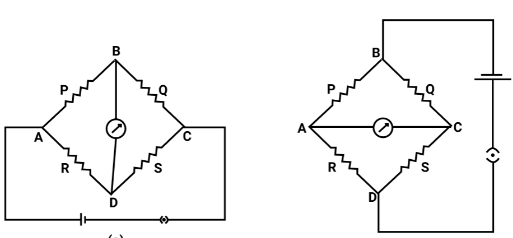चित्र (a) में संतुलन की स्थिति में $\frac{P}{Q} = \frac{R}{S}$ चित्र (b) में संतुलन की स्थिति में $\frac{R}{P} = \frac{S}{Q}{{\;}}$अथवा$\frac{R}{S} = \frac{P}{Q}$ 11.${\mathbf{8}}{\text{ }}{\mathbf{V}}$विद्युत वाहक बल की एक संचायक बैटरी जिसका आन्तरिक प्रतिरोध ${\mathbf{0}}.{\mathbf{5}}{\text{ }}\Omega$है, को श्रेणीक्रम में ${\mathbf{15}}.{\mathbf{5}}{\text{ }}\Omega$के प्रतिरोधक का उपयोग करके ${\mathbf{120}}{\text{ }}{\mathbf{V}}$के ${\mathbf{D}}.{\mathbf{C}}.$स्रोत द्वारा चार्ज किया जाता है। चार्ज होते समय बैटरी की टर्मिनल वोल्टता क्या है? चार्जकारी परिपथ में प्रतिरोधक को श्रेणीक्रम में सम्बद्ध करने का क्या उद्देश्य है? हल: जब बैटरी को $120{\text{ }}V$की $D.C.$सप्लाई से आवेशित किया जाता है, तो बैटरी में सामान्य अवस्था की अपेक्षा धारा विपरीत दिशा में होगी। अतः बैटरी की टर्मिनल वोल्टता, $V{\text{ }} = {\text{ }}E{\text{ }} + {\text{ }}Ir$ यहाँ विद्युत वाहक बल, $E{\text{ }} = {\text{ }}8{\text{ }}V$, आन्तरिक प्रतिरोध $r{\text{ }} = {\text{ }}0.5{\text{ }}\Omega$ परिपथ में धारा, = \frac{{120 - 8}}{{15.5 + 0.5}} = \frac{{112}}{{16}} = 7\;A V = 8 + 7 \times 0.5 = 11.5\;{\text{V}}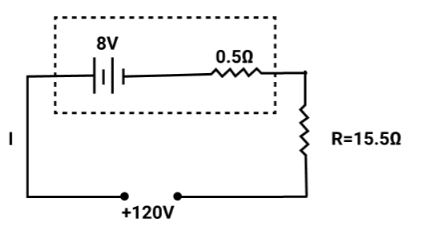श्रेणी-प्रतिरोध बाह्य $D.C.$सप्लाई से ली गई धारा को सीमित करता है। बाह्य प्रतिरोध की अनुपस्थिति में संचायक बैटरी द्वारा अनुमेय सुरक्षित धारा के मान से अधिक धारा प्रवाहित हो सकती है। 12. किसी पोटेशियोमीटर व्यवस्था में, ${\mathbf{1}}.{\mathbf{25}}{\text{ }}{\mathbf{V}}$विद्युत वाहक बल से एक सेल का सन्तुलन बिन्दु तार के ${\mathbf{35}}.{\mathbf{0}}{\text{ }}{\mathbf{cm}}$लम्बाई पर प्राप्त होता है। यदि इस सेल को किसी अन्य सेल द्वारा प्रतिस्थापित कर दिया जाए तो सन्तुलन बिन्दु ${\mathbf{63}}.{\mathbf{0}}{\text{ }}{\mathbf{cm}}$पर स्थानान्तरित हो जाता है। दूसरे सेल का विद्युत वाहक बल क्या है ? हल: दिया है सेल {E_1} = 1.25\;V के लिए अविक्षेप बिन्दु की दूरी {l_1} = 35.0\;cm {E_2} = ?, जबकि {l_2} = 63.0\;cm विभवमापी के लिए E \propto l \therefore \;\frac{{{E_2}}}{{{E_1}}} = \frac{{{l_2}}}{{{l_1}}} \Rightarrow \;{E_2} = \frac{{{l_2}}}{{{l_1}}} \times {E_1} = \frac{{63.0}}{{35.0}} \times 1.25\;V = 2.25\;V अत: दूसरे सेल का वि० वा० बल {E_2} = 2.25\;V 13.किसी ताँबे के चालक में मुक्त इलेक्ट्रॉनों का संख्या घनत्व ${\mathbf{8}}.{\mathbf{5}}{\text{ }}{\mathbf{x}}{\text{ }}{\mathbf{1}}{{\mathbf{0}}^{{\mathbf{28}}}}{{\mathbf{m}}^{\mathbf{3}}}$आकलित किया गया है। ${\mathbf{3}}{\text{ }}{\mathbf{m}}$लम्बे तार के एक सिरे से दूसरे सिरे तक अपवाह करने में इलेक्ट्रॉन कितना समय लेता है? तार की अनुप्रस्थ-काट ${\mathbf{2}}.{\mathbf{0}}{\text{ }}{\mathbf{x}}{\text{ }}{\mathbf{1}}{{\mathbf{0}}^{ - {\mathbf{6}}}}\;{{\mathbf{m}}^{\mathbf{2}}}$है और इसमें ${\mathbf{3}}.{\mathbf{0}}{\text{ }}{\mathbf{A}}$धारा प्रवाहित हो रही है। हल: दिया है, इलेक्ट्रॉन का संख्या घनत्व $n{\text{ }} = {\text{ }}8.5{\text{ }}x{\text{ }}{10^{28}}{m^3}$ तार की लम्बाई $l{\text{ }} = {\text{ }}3{\text{ }}m$ तार के अनुप्रस्थ काट का क्षेत्रफल $A{\text{ }} = {\text{ }}20{\text{ }}x{\text{ }}{10^{ - 6}}\;{m^2}$ तार में धारा $i{\text{ }} = {\text{ }}3.0{\text{ }}A$ इलेक्ट्रॉन का आवेश $e{\text{ }} = {\text{ }}1.6{\text{ }}x{\text{ }}{10^{ - 19}}C$ माना तार के एक (UPBoardSolutions.com) सिरे से दूसरे सिरे तक प्रवाहित होने में इलेक्ट्रॉन द्वारा लिया गया समय t है, तब सूत्र $i{\text{ }} = {\text{ }}neA{v_d}$से, {v_d}\;\; = i/neA\;\;\; = \frac{3}{{8.5 \times {{10}^{28}} \times 1.6 \times {{10}^{ - 19}} \times 2.0 \times {{10}^{ - 6}}}}\;m{s^{ - 1}}\;\;\; = 1.103 \times {10^{ - 4}}\;m{s^{ - 1}}\; t\;\; = \frac{l}{{{v_d}}} = \frac{3}{{1.103 \times {{10}^{ - 4}}}}\;\;\; = 2.72 \times {10^4}सेकण्ड = 7घण्टे33 मिनट 14. पृथ्वी के पृष्ठ पर ऋण|त्मक पृष्ठ आवेश घनत्व {10^{ - 9}}{\text{Cc}}{{\text{m}}^{ - 2}} है। वायुमंडल के ऊपरी भाग और पृथ्वी के बीच 400{\text{kV}} विभवांतर (नीचे के वायुमंडल की कम चालकता के कारण ) के परिणामतः समुच पृथ्वी पर केवल 1800\;{\text{A}} की धारा है। यदि वायुमंडलीय विद्युत क्षेत्र बनाए रखने हेतु कोई प्रक्रिया न हो तो पृथ्वी के पृष्ठ को उदासीन करने हेतु लगभग) कितना समय लगेगा? ( व्यावहारिक रूप से यह कभी नहीं होता है क्योंकि विद्युत आवेशों की पुनः पूर्ति की एक प्रक्रिया है तथा पृथ्वी के विभित्र भागों में लगातार तड़ित झंझा एवं तड़ित का होना) (पृथ्वी की त्रिज्या = 6.37 \times {10^6}\;{\text{m}} ) उत्तर: दी गई जानकारी पृथ्वी की त्रिज्या {\text{RE}} = 6.37 \times {10^6}\;{\text{m}}, प्रिष्ठिय आवेश घनत्व = \sigma = {10^{ - 19}}{\text{C}}/{\text{c}}{{\text{m}}^2} = {10^{ - 5}}{\text{C}}/{{\text{m}}^2} वायुमंडल से पृथ्वी पर धारा = 1800\;{\text{A}} पृथ्वी की सतह पर कुल आवेश = q = प्रिश्ठिय क्षेत्रफल x \times \sigma = 4\pi R{E^2} पृथ्वी के निरावेशन में लगा समय t = ? {\text{t}} = \frac{{\text{q}}}{{\text{i}}}{\text{ }} = \frac{{4 \times 3.14 \times {{\left( {6.37 \times {{10}^6}} \right)}^2} \times {{10}^{ - 5}}}}{{1800}} = 283\;{\text{s}} 15: 1. $6$ लेड एसिड संचायक सेलों को जिनमें प्रत्येक का विद्युत वाहक बल 2\;V तथा आंतरिक प्रतिरोध 0.015\;\Omega है जो इसके साथ श्रेणी संबद्ध है, में धारा की आपूर्ति के लिए किया जाता है। बैटरी से कितनी धारा ली गई है एवं इसकी टर्मिनल वोल्टता क्या है? उत्तर . दी गई जानकारी E = 2.0\;{\text{V}},{\text{n}} = 6,{\text{r}} = 0.015\;\Omega ,\;{\text{R}} = 8.5\;\Omega सेल जब श्रेणीक्रम में हो तब धारा, {\text{I}} = \frac{{{\text{nE}}}}{{{\text{R}} + {\text{nr}}}} I = \frac{{6 \times 2.0}}{{\{ (8.5) + (6 \times 0.015)\} }} = \frac{{12}}{{8.59}} = 1.4\;{\text{A}} टर्मिनल वोल्टता V = IR = 1.4 \times 8.5 = 11.9\;V 1. एक लंबे समय तक उपयोग में लाए गए संचयक सेल का विद्युत वाहक बल 1.9\;{\text{V}} और विशाल आंतरिक प्रतिरोध 380\;\Omega है । सेल से कितनी अधिकतम धारा ली जा सकती है? क्या सेल से प्राप्त यह धारा किसी कर की प्रवर्तक मोटर को स्टार्ट करने में सक्षम होगी? अधिकतम धारा R = 0 सेल से ली गई धारा I = \frac{E}{{(R + r)}} अधिकतम धारा = {1_{\max }} = \frac{{\text{E}}}{{\text{r}}} = (\frac{{1.9}}{{380}}){\text{A}} = 0.005\;{\text{A}} सेल कार की प्रवर्तक मोटर को चलाने में सक्षम नहीं है क्योंकि कर की प्रवर्तक मोटर को चलाने के लिए बहुत अधिक धारा लगभग 100\;{\text{A}} की आवश्यकता होती है। 16: दो समान लंबाई की तारों में एक एलुमिनियम के तारों को क्यों पसंद किया जाता है {\left( {{{\text{p}}_{{\text{Al}}}} = 2.63 \times {{10}^{ - 8}}\;\Omega m,{\rho _{{\text{cu}}}} = 1.72 \times 10} \right.^{ - 8}}\;\Omega m . Al का आपेक्षिक घनत्व = 2.7, कॉपर का आपेक्षिक घनत्व = 8.9) उत्तर. दी गई जानकारी अनुसार \rho {\text{Al}} = {\text{I}}/{\text{A}}({\text{Al}}) = {\rho _{{\text{Cu}}}} = \frac{{\text{I}}}{{{\text{A}}({\text{CU}})}} = \frac{{{{\text{A}}_{{\text{Al}}}}}}{{{A_{C{\text{u}}}}}} = \frac{{{\rho _{{\text{Al}}}}}}{{\rho {}_{C{\text{u}}}}} यदि हम माने कि {\text{d}}_{{\text{Al}}}^{} जेडएक्स तथा {{\text{d}}_{{\text{Cu}}}}, Al तथा Cu के घनत्व है तो, उनके द्रव्यमानों का अनुपात होगा \frac{{{{\text{m}}_{{\text{Al}}}}}}{{{{\text{m}}_{{\text{Cu}}}}}} = ({A_{{\text{Al}}}} \times I \times {d_{Al}})/({A_{Cu}} \times I \times {d_{Cu}}) $= (\frac{{{\rho _{Al}}}}{{\rho {}_{Cu}}}) \times (\frac{{{d_{Al}}}}{{d{}_{Cu}}})$ = \frac{{m_{Al}^{}}}{{{m_{Cu}}}} = \left\{ {\frac{{\left( {2.63 \times {{10}^{ - 8}}} \right)}}{{\left( {1.72 \times {{10}^{ - 8}}} \right)}}} \right\} \times (\frac{{2.7}}{{8.9}}) = 0.46 = \frac{1}{2} उपूर्युक्त उत्तर से स्पष्ट है कि अल्युमिनियम के तार का द्रव्यमान , कॉपर के तार के द्रव्यमान का आधा है अर्थात अल्युमिनियम का तार उत्तर का है । इसी कारण ऊपर से जाने वाले बिजली के केबिलों में एलुमिनियम के तारों का प्रयोग किया जाता है।यदि कॉपर के तारों का प्रयोग किया जाए तो खंबे और अधिक मजबूत बनाने होंगे। 17: मिश्रातु मैंगनिन के बने प्रतिरोधक पर लिए गए निम्नलिखित प्रेक्षणों से आप क्या निष्कर्ष निकाल सकते हैं?  धाराA वोल्टेजV धाराA वोल्टेजV ${\mathbf{0}}.{\mathbf{2}}$ $3.94$ $\begin{array}{*{20}{l}}{3.0}\end{array}$ $\begin{array}{*{20}{l}}{59.2}\end{array}$ $0.4$ $7.87$ $4.0$ $78.8$ $0.6$ $\begin{array}{*{20}{l}}{11.8} \end{array}$ $5.0$ $98.6$ $0.8$ $15.7$ $6.0$ $\begin{array}{*{20}{l}}{118.5} \end{array}$ $\begin{array}{*{20}{l}}{1.0} \end{array}$ $\begin{array}{*{20}{l}}{19.7} \end{array}$ $\begin{array}{*{20}{l}}{7.0} \end{array}$ $\begin{array}{*{20}{l}}{138.2} \end{array}$ $\begin{array}{*{20}{l}}{2.0} \end{array}$ $\begin{array}{*{20}{l}}{39.4} \end{array}$ $\begin{array}{*{20}{l}}{8.0} \end{array}$ $\begin{array}{*{20}{l}}{158.0} \end{array}$ उत्तर. दी गई सारणी का निरीक्षण करने से यह स्पष्ट है कि $\;19.7{\text{ }}\Omega$ इससे यह स्पष्ट होता है कि मैंगनिन का प्रतिरोधक लगभग पूरे वोल्टेज परिसर में ओह्म के नियम का पालन करता है इसका अर्थ है कि मैंगनिन की प्रतिरोधकता पर ताप का बहुत कम प्रभाव पड़ता है। 18: निम्नलिखित प्रश्नों के उत्तर दीजिए- 1. किसी असमान अनुप्रस्थ काट वाले धाल्विक चालक से एकसमान धारा प्रवाहित होती है। निम्नलिखित में से चालक में कौनसी अचर रहती है धारा, धारा घनत्व, विद्युत क्षेत्र, अपवाह चाल। उत्तर: जैसा कि दिया गया है, केवल धारा अचर रहती है। अन्य राशियां अनुप्रस्थ क्षेत्रफल के व्युत्क्रमानुपाती हैं। 1. क्या सभी परीपथिय अवयवों के लिए ओह्म का नियम सार्वत्रिक रूप से लागू होता है? यदि नहीं, तो उन अस व्यवो के उदाहरण दीजिए जो ओह्म के नियम का पालन नहीं करते। उत्तर: नहीं, ओह्म का नियम सभी परिपथिय अवयवोंर नहीं लागू होता।अर्द्ध चालक युक्तियां जैसे संधि डायोड तथा ट्रांजिस्टर तथा निर्वात नलिकाएं जैसे डायोड, ट्रायोड वाल्व इसी प्रकार की युक्तियां है। 1. किसी निम्न वोल्टता संभरण जिससे उच्च धारा देनी होती है, आंतरिक प्रतिरोध बहुत कम होना चाहिए ? क्यों? उत्तर: किसी दंभरण से प्राप्त अधिकतम धारा ${I_{\max }} = \frac{{\text{E}}}{{\text{r}}}$ वि. वा. बल कम है इसलिए पर्याप्त धारा प्राप्त करने के लिए आंतरिक प्रतिरोध कम होना चाहिए। दूसरा कारण यह है कि प्रतिरोध अधिक होने से सेल द्वारा दी गई ऊर्जा का अधिकांश भाग सेल के भीतर ही व्यय हो जाता है। 1. किसी उच्च विभव (H.T.) संभरन, मान लीजिए 6{\text{kV}}, का आंतरिक प्रतिरोध अत्यधिक होना चाहिए? क्यों? उत्तर: यदि आंतरिक बल कम होगा तो किसी कारण लघु पथित होने की दशा में संभरण से अति उच्च धारा प्रवाहित होगी तथा संभरण के क्षतिग्रस्त होने की संभावना उत्पन्न हो सकती है। 19: सही विकल्प छांटिए 1. धातुओं की मिश्रातुओं की प्रतिरोधकता प्राय उनकी अवयव धातुओं की अपेक्षा (अधिक/कम) होती है। उत्तर: अधिक 1. आमतौर पर मिश्रातुओं के प्रतिरोध का ताप गुणांक, शुद्ध धातुओं के प्रतिरोध के ताप गुणांक से बहुत (कम/अधिक) होती है। उत्तर: कम 1. मिश्रातु मैंगनिन की प्रतिरोधकता ताप में वृद्धि के साथ लगभग स्वतंत्र है/ तेजी से बढ़ती है) उत्तर: स्वतंत्र है। 1. किसी प्रारुपी विद्युत् रोधी (उदाहरणार्थ, अंबर) की प्रतिरोधकता की तुलना में \left( {{{10}^{22}}/{{10}^{23}}} \right) कोटि के गुणांक से बड़ी होती है। उत्तर: {10^{22}} 20: 1. आपको {\text{R}} प्रतिरोध वाले n प्रतिरोधक दिए गए हैं।(i) अधिकतम (ii) न्यूनतम प्रभावी प्रतिरोध प्राप्त करने के लिए आप इन्हे किस प्रकार संयोजित करेंगे? अधिकतम और न्यूनतम प्रतिरोधी का अनुपात क्या होगा? उत्तर: .(a) (i) प्रतिरोधी को श्रेणी में जोड़ने पर अधिकतम प्रतिरोध प्राप्त होता है । श्रेणीक्रम में तुल्य प्रतिरोध Rs = R + R + R + \ldots \ldots \ldots \ldots ..n पद $= n{\text{ }}R$ (ii) न्यूनतम प्रतिरोध प्राप्त करने के लिए इन्हे पार्व्व क्रम में जोड़ना होगा। $\frac{1}{{{{\text{R}}_{\text{p}}}}} = \frac{1}{R} + \frac{1}{R} + \ldots \ldots \ldots .{\text{n}}$ पद = \frac{{\text{n}}}{R} तुल्य प्रतिरोध (पार्श्व क्रम में) {R_p} = \frac{R}{n} अभीष्ट अनुपात $\frac{{{{\text{R}}_{\text{s}}}}}{{{{\text{R}}_{\text{p}}}}} = \frac{{{\text{nR}}}}{{(\frac{{\text{R}}}{{\text{n}}})}} = \frac{{{{\text{n}}^2}}}{1}$ = {R_s}:{R_p} = {n^2}:1 1. यदि $1$ ओह्म, $2$ ओह्म , $3$ ओह्म के प्रतिरोध दिए गए हो उनको आप किस प्रकार संयोजित करेंगे? की प्राप्त तुल्य प्रतिरोध हों: (i) $\left( {\frac{{11}}{3}} \right){\text{ }}\Omega$, (ii) $\left( {\frac{{11}}{5}} \right)\;\Omega$ , (iii) $6\;\Omega$ , (iv) $\left( {\frac{6}{{11}}} \right)\;\Omega$? (b) दिए गए $3$ प्रतिरोध $1$ ओह्म, $2$ ओह्म, $3$ ओह्म है। इसलिए ${R_1} = 1\;\Omega ,{\text{ }}{R_2} = 2\;\Omega ,{\text{ }}{R_3} = 3{\text{ }}\Omega$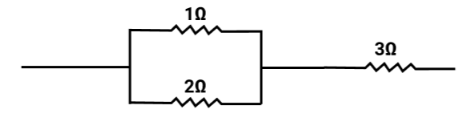(i) $\left( {\frac{{11}}{3}} \right){\text{ }}\Omega$ का प्रतिरोध प्राप्त करने के लिए {{\text{R}}_1},{{\text{R}}_2} को पार्श्व क्रम में व {{\text{R}}_3} को श्रेणी में जोड़ना होगा। {\text{R }} = \left\{ {\frac{{{{\text{R}}_1}{{\text{R}}_2}}}{{\left( {{{\text{R}}_1} + {{\text{R}}_2}} \right)}}} \right\} + {{\text{R}}_3} = \{ \frac{{(1 \times 2)}}{{(1 + 2)}}\} + 3 = \frac{{11}}{3}\;\;\Omega (ii) $\left( {\frac{{11}}{5}} \right)\;\Omega$ का प्रतिरोध प्राप्त करने के लिए {{\text{R}}_2},{{\text{R}}_3} को पार्व्व क्रम में तथा {{\text{R}}_1} को श्रेणी में जोड़ना होगा। {\text{R}} = \{ \frac{{(2 \times 3)}}{{(2 + 3)}}\} + 1 = (\frac{6}{5}) + 1 = \frac{{11}}{5}\;\Omega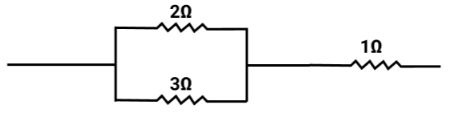(iii) 6 ओह्म का प्रतिरोध प्राप्त करने के लिए तीनों प्रतिरोधी को श्रेणी क्रम में जोड़ना होगा। तब {\text{ Req }} = {R_1} + {R_2} + {R_3} = 1 + 2 + 3 = 6{\text{ ohm }} (iv) $\left( {\frac{6}{{11}}} \right)\;\Omega$ का प्रतिरोध प्राप्त करने के लिए तीनों को पार्व्व क्रम में जोड़ना होगा। $1/{\text{Re}} = (\frac{1}{1}) + (\frac{1}{2}) + (\frac{1}{3}) = \frac{{(6 + 3 + 2)}}{6} = \frac{{11}}{6}$ ohm $R = \left( {\frac{6}{{11}}} \right)\;\Omega$ 1. चित्र में दिखाए गए नेटवर्कों का तुल्य प्रतिरोध प्राप्त कीजिए।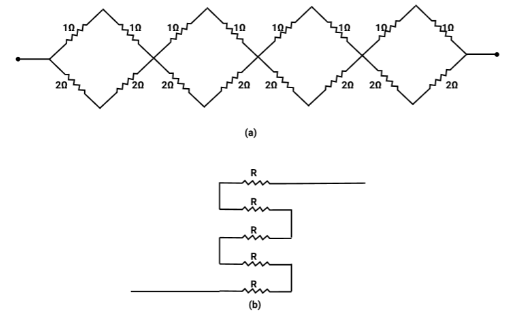(c) (i) प्रत्येक पॉश में 1\Omega - 1\Omega श्रेणीक्रम में तथा 2\;\Omega - 2\;\Omega श्रेणीक्रम में हैं। इं शाखाओं के अलग अलग प्रतिरोध 1 + 1 = 2\;\Omega व 2 + 2 = 4\;\Omega ये दो शाखाएं अब समांतर क्रम में जुड़ी हैं। प्रत्येक पॉश का प्रतिरोध $= \frac{{(2 \times 4)}}{{(2 + 4)}} = \frac{8}{6} = \frac{4}{3}\;\Omega$ इस प्रकार के चर पॉश श्रेणी क्रम में जुड़े हैं। नेटवर्क का प्रतिरोध R$$ = \frac{4}{3} + \frac{4}{3} + \frac{4}{3} + \frac{4}{3} = \frac{{16}}{3}\;\Omega$

(b) R ohm के $5$ प्रतिरोध श्रेणीक्रम में जुड़े हैं।

नेटवर्क का प्रतिरोध $R$$= R + R + R + R + R = 5\;\Omega 21: किसी 0.5 ओह्म आंतरिक प्रतिरोध वाले 12 \vee के एक संभरण (supply) से दिए गए चित्र में दर्शाए गए अनंत नेटवर्क द्वारा ली गई धारा का मान ज्ञात कीजिए। प्रत्येक का प्रतिरोध मान 1 ओह्म है।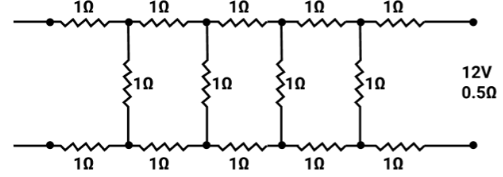उत्तर. यदि माना कि बिंदुओं A तथा B के तुल्य प्रतिरोध R है। अनन्त \pm 1 = अनन्त , अतः बिंदुओं C व D के बीच प्रतिरोध वहीं होगा जो बिंदुओं A व B के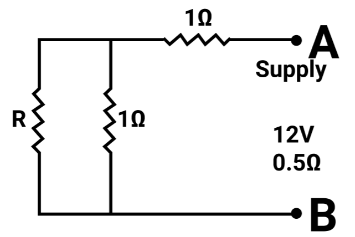बीच है, इसलिए समांतर क्रम में जुड़े प्रतिरोध R तथा 1{\text{ohm}} का तुल्य प्रतिरोध$${R_1} = \frac{{(R \times 1)}}{{(R + 1)}} = \frac{R}{{R + 1}}$$बिन्दु$A$तथा$B$के बीच तुल्य प्रतिरोध$R(AB) = {R_1} + 1 + 1$परिक्सलपना से${{\text{R}}_1} + 1 + 1 = {\text{R}}(\frac{R}{{R + 1}}) + 2 = RR + 2(R + 1) = R(R + 1)3R + 2 = {R^2} + R{R^2} - 2R - 2 = 0$$R = \frac{{2 \pm {{\{ (4 + 4) + 2\} }^{\frac{1}{2}}}}}{2} = \frac{{2 \pm {{(12)}^{\frac{1}{2}}}}}{2} = 1 \pm {(3)^{\frac{1}{2}}}$ ऋण|त्मक प्रतिरोध नहीं हो सकता${\text{R}} = \left\{ {1 + {{(3)}^{\frac{1}{2}}}} \right\} = (1 + 1.732) = 2.732\;\Omega $। (सप्लाई से ली गई धारा)$ = \frac{{12}}{{(2.732 + 0.5)}} = \frac{{12}}{{3.3242}}\;{\text{A}} = 3.7\;\Omega $22: चित्र में एक पोटेंशियोमीटर दर्शाया गया गया है जिसमें एक$2.0\;{\text{V}}$और आंतरिक प्रतिरोध$0.40$ओह्म का कोई सेल, पोटेंशियोमीटर के प्रतिरोधक तार$A B$पर वोल्टता पात बनाए रखता है। कोई मानक सेल जो$1.02\;{\text{V}}$का अचर विद्युत वाहक का बल बनाए रखता है ककछ${\text{mA}}$की बहुत सामान्य धाराओं के लिए ) तार की$67.3\;{\text{cm}}$लंबाई पर संतुलन बिन्दु होता देता है। मानक सेल सीटी न्यून धारा लेना सुनिश्चित करने के लिए इसके साथ परिपथ में श्रेणी$600{\text{k}}$ओह्म का एक अति उच्च प्रतिरोध इसके साथ संबद्ध किया जाता है, जिसके संतुलन बिन्दु प्राप्त होने के निकट लघु पथित (shorted) कर दिया जाता है। इसके बाद मानक सेल को किसी अज्ञात विद्युत वाहक बल$\varepsilon $के सेल से प्रस्थापित कर दिया जाता जिससे संतुलन बिन्दु तार की$82.3\;{\text{cm}}$लंबाई पर प्राप्त होता है। 1.$\varepsilon $का मान क्या है?$ = 1.02\;{\text{V}}$उत्तर: (a) दो सेलों के बलो की तुलना करने सूत्र $\frac{{{E_2}}}{{{E_1}}} = \frac{{{I_2}}}{{{I_1}}}$ $\frac{E}{{Es}} = \frac{1}{{Is}}$$E = \frac{{(\frac{1}{{{I_{\text{s}}}}})}}{{{{\text{E}}_{\text{s}}}}}{E_s}$प्रामाणिक सरल का वै. वा. बल${I_s}$प्रामाणिक सेल संतुलन की लंबाई=$67.3\;{\text{cm}}$। = अज्ञात बै वा बल के दिल से संतुलन की लम्बाई$ = 82.3\;{\text{cm}}$अज्ञात वे वा. बल$E{\text{ }} = \{ \frac{{(82.3\;{\text{cm}})}}{{(67.3\;{\text{cm}})}}\}  \times 1.02\;{\text{V}} = 1.25\;{\text{V}}$1.$600{\text{kohm}}$के उच्च प्रतिरोध का क्या प्रयोजन है? धारा मापी में धारा को कम करने के लिए उच्च प्रतिरोध की आवश्यकता होती है जब जौकी संतुलन बिन्दु से दूर है।इस कारण प्रामाणिक सेल हानि से बचा रहता है। 1. क्या इस उच्च प्रतिरोध से संतुलन बिन्दु प्रभावित होता है? संतुलन की स्थिति में सेल के द्वितीयक परिपथ में धारा नहीं बहती इसलिए संतुलन बिन्दु उच्च प्रतिरोध से प्रभावित नहीं होता। 1. उपरोक्त स्थिति में यदि पोटेंशियोमीटर के परिचालक सेल का विद्युत वाहक बल$2.0\;{\text{V}}$के स्थान पर$1.0\;{\text{V}}$हो तो क्या यह विधि फिर भी सफल रहेगी ? परिचारक सेल के आंतरिक प्रतिरोध से संतुलन बिंदु प्रभावित नहीं होता क्योंकि तार पर विभव प्रणवता पहले से ही नियत रख दी गई है। 1. क्या यह परिपथ कुछ${\text{mV}}$की कोटि के अत्यल्प विद्युत वाहक बलों (जैसे कि किसी प्रारूपी ताप वैध्यू युग्म का विद्युत वाहक बल) के निर्धारण में सफल होगी? यदि नहीं, तो आप इसमें किस प्रकार संशोधन करेंगे? नहीं, क्योंकि जब परिचारक सेल का वे. वि. बल, द्वितीयक परिपथ के सेल के वै. वि. बल,$E$से अधिक होगा तब विभव मापी कार्य कर पाएगा।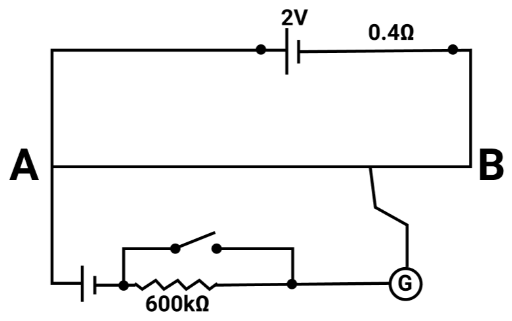1. संतुलन बिन्दु सिरे${\text{A}}$के निकट होगा तो मापन में बहुत त्रुटि होती है। इसलिए परिचारक सेल के श्रेणीक्रम में एक परिवर्ती प्रतिरोध$R$जोड़ते हैं तथा इसका मान इस प्रकार व्यवस्थित किया जाता है कि तार$AB$के सिरों के बीच विभवपात द्वितीयक सेल से थोड़ा ही अधिक हो जिससे संतुलन बिन्दु अधिक लंबाई पर प्राप्त हो, इस प्रकार मापन में त्रुटि कम होगी।मापन की यथार्थता भी बढ़ेगी। 23: चित्र में किसी$1.5 \vee$के सेल का आंतरिक प्रतिरोध मापने के लिए एक$2.0\;{\text{V}}$का पोटेंशियोमीटर दर्शाया गया है। खुले परिपथ में सेल का संतुलन बिन्दु$76.3\;{\text{cm}}$पर मिलता है। सेल के बाह्य परिपथ में$9.5$ओह्म प्रतिरोध का एक प्रतिरोधक संयोजित करने पर संतुलन बिन्दु पोटेंशियोमीटर के तार की$64.8\;{\text{cm}}$लंबाई पर पहुंच जाता है। सेल के आंतरिक प्रतिरोध का मान ज्ञात कीजिए।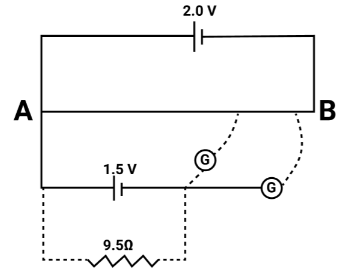उत्तर. माना कुजियां${{\text{K}}_1},\;{{\text{K}}_2}$है तथा इन कुजियों को क्रमशः बंद करके विभवमापी के तार का संतुलन बिन्दु प्राप्त करने पर यदि संगत लंबाई क्रमशः${I_1},{I_2}$हो तो सिरों$R$का विभवान्टर$ = k{l_2} = X\mid $$I =$ विभव मापी के तार में धारा$K = $विभव की प्रवणता ]$\frac{{{\text{K}}{{\text{I}}_1}}}{{{\text{K}}{{\text{I}}_2}}} = \frac{{{\text{RI}}}}{{{\text{XI}}}} = \frac{{\text{R}}}{{\text{X}}} = \frac{{{{\text{I}}_1}}}{{{{\text{I}}_2}}}{\text{X}} = {\text{R}}\left( {\frac{{{{\text{I}}_2}}}{{{{\text{I}}_1}}}} \right){\text{R}} = 10.0\Omega ,{{\text{l}}_1} = 58.3\;{\text{cm}},{I_2} = 68.5\;{\text{cm}}{\text{X}} = 10\;\Omega [\frac{{68.5\;{\text{cm}}}}{{58.3\;{\text{cm}}}}] = 11.75{\text{ohm}}$यदि संतुलन बिन्दु प्राप्त नहीं होता तो इससे यह स्पष्ट होता है कि$R$या$x$के सिरों के बीच विभवांतर विभव मापी के तार$AB$के सिरों के बीच विभवांतर से अधिक है। ऐसी स्थिति में बाह्य परिपथ में धारा का मान कम करने केलिए श्रेणी क्रम में एक उचित प्रतिरोध जोड़ने की आवश्यक्ता है जो बिन्दु$C$व$D\$ के बीच जोड़ा जाएगा।

### NCERT Solutions for Class 12 Physics Chapter 3 Current Electricity in Hindi

Chapter-wise NCERT Solutions are provided everywhere on the internet with an aim to help the students to gain a comprehensive understanding. Class 12 Physics Chapter 3 solution Hindi medium is created by our in-house experts keeping the understanding ability of all types of candidates in mind. NCERT textbooks and solutions are built to give a strong foundation to every concept. These NCERT Solutions for Class 12 Physics Chapter 3 in Hindi ensure a smooth understanding of all the concepts including the advanced concepts covered in the textbook.

NCERT Solutions in Hindi medium have been created keeping those students in mind who are studying in a Hindi medium school. These NCERT Solutions for Class 12 Physics Current Electricity in Hindi medium pdf download have innumerable benefits as these are created in simple and easy-to-understand language. The best feature of these solutions is a free download option. Students of Class 12 can download these solutions at any time as per their convenience for self-study purpose.

These solutions are nothing but a compilation of all the answers to the questions of the textbook exercises. The answers/solutions are given in a stepwise format and very well researched by the subject matter experts who have relevant experience in this field. Relevant diagrams, graphs, illustrations are provided along with the answers wherever required. In nutshell, NCERT Solutions for Class 12 Physics in Hindi come really handy in exam preparation and quick revision as well prior to the final examinations.

## FAQs on NCERT Solutions for Class 12 Physics Chapter 3 Current Electricity in Hindi

1. How to solve the problems present in Chapter 3 Current Electricity of Class 12 Physics faster?

The first step to being able to solve Physics problems is to learn the concepts. Then, a student should learn the formula related to each concept. Learning the derivations is also an important part of learning formulas. If the students do these, then they will be able to solve their Chapter 3 Current Electricity of Class 12 Physics problems faster. The main focus of the students should definitely be on the concepts. Once the students learn the concepts, then it will become really easy for them to understand a problem and what the answer demands. In this way, the students will very easily be able to solve any problem present in NCERT Solutions for Chapter 3 Current Electricity of Class 12 Physics.

2. What are the key benefits of NCERT Solutions for Chapter 3 Current Electricity of Class 12 Physics in Hindi?

The key benefits of NCERT Solutions for Chapter 3 Current Electricity of Class 12 Physics in Hindi are as follows-

• The answers are all accurate.

• The solutions help the students with the answering pattern of NCERT.

• The answers help the students revise whole chapters before exams.

• The solutions all follow the CBSE guidelines thoroughly.

• The solutions are well framed and easy to understand.

• The solutions help students answer a question in a more accurate manner.

3. Is Chapter 3 Current Electricity of Class 12 Physics in Hindi difficult?

Class 12 Physics is moderately easy. Students might face difficulty if they do not understand the concepts of Physics. Once the concepts are clear, the subject is fairly easy. As I have also mentioned previously, the key to making Physics easy to learn is to focus on the concepts. Once you are clear and thorough with the concepts, then Physics will definitely seem easy as well as interesting to you.

4. How many chapters are there in 12th NCERT Physics apart from Chapter 3?

There are a total of 15 chapters in Class 12 NCERT Physics. These fifteen chapters consist of various topics. There are several units in the Class 12 NCERT Physics book. Under these units, the chapters are arranged according to the topics.

For a better understanding of each chapter and each topic, the students can refer to the Vedantu website. This website provides the students with all the solutions for their Class 12 NCERT Physics.

5. What are the important chapters of Class 12th NCERT Physics apart from Chapter 3?

All the chapters of Class 12 Physics are important. Many chapters are also interrelated which means that you would not understand one chapter if you skip any previous chapters. So, the importance of all the chapters is equal. Students should be careful not to skip any of the chapters in case they miss out on important concepts. All the chapters will carry a certain weightage in their examinations.SHARETWEETSHARESUBSCRIBE